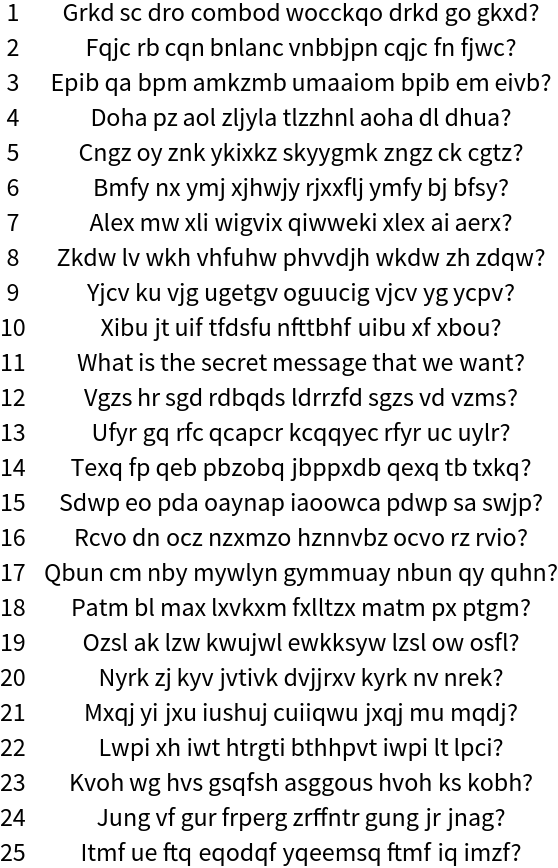#Function Repository Resource:

# CaesarDecipher

Decipher a Caesar-enciphered string

Contributed by: Sander Huisman
 ResourceFunction["CaesarDecipher"][string,n] deciphers a Caesar-enciphered string that was enciphered by shifting letters in string by n places further in the Latin alphabet. ResourceFunction["CaesarDecipher"][{s1,s2,…},n] deciphers each string si. ResourceFunction["CaesarDecipher"][n] represents an operator form of ResourceFunction["CaesarDecipher"] that can be applied to an expression.

## Details and Options

CasearDecipher replaces each plain text character with a different one a fixed number of places n up the alphabet. As an example, here we show it for n=3: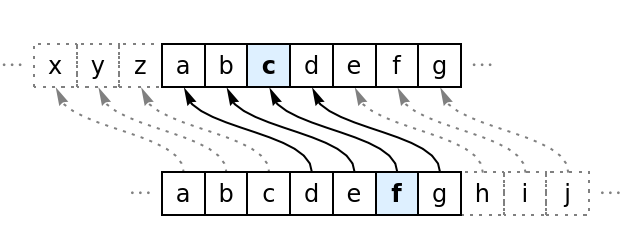The letter f is converted to the letter c.

ResourceFunction["CaesarDecipher"][string] performs the ROT13 substitution decipher.
n can be negative, shifting the letters to the right.
ResourceFunction["CaesarDecipher"] lists over the first argument.
Letters retain their case (lowercase/uppercase).

## Examples

### Basic Examples

Perform a Caesar decipher with a shift of 2:

 In:=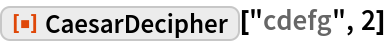Out=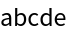Decipher a text by shifting the letters 9 positions back:

 In:=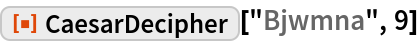Out=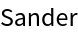### Scope

Cipher/decipher using a shift of n=13, also known at the ROT13 cipher:

 In:=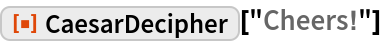Out=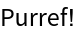In:=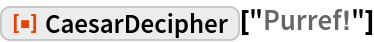Out=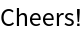Decipher multiple strings:

 In:=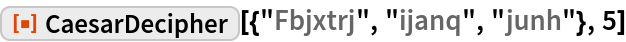Out=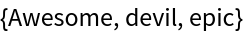Create an operator form of the function that deciphers with a shift of 5:

 In:=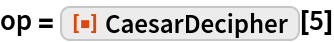Out=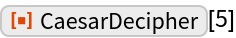Use the operator:

 In:=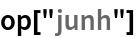Out=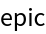### Applications

Decipher a message:

 In:=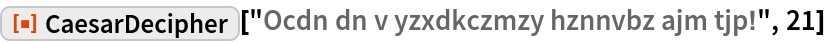Out=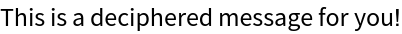Decipher a message:

 In:=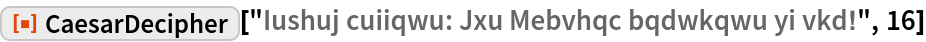Out=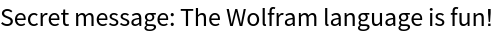### Properties and Relations

Enciphering can be done using the deciphering function but with a negative shift:

 In:=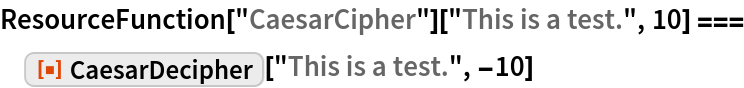Out=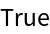If the shift is an integer multiple of 26, the Caesar decipher does not affect the input string:

 In:=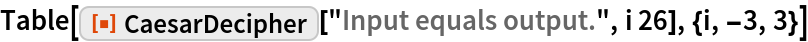Out=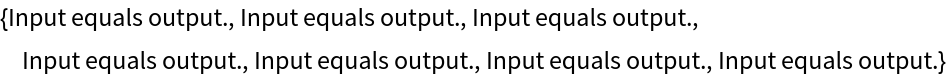### Neat Examples

Try out all 25 options to decipher a message and find the original message:

 In:=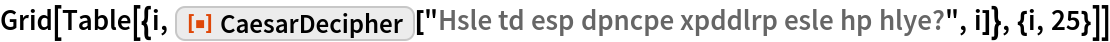Out=